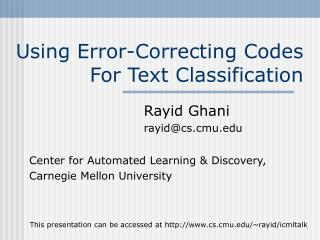DownloadDownload PresentationUsing Error-Correcting Codes For Text Classification

# Using Error-Correcting Codes For Text Classification

Télécharger la présentation## Using Error-Correcting Codes For Text Classification

- - - - - - - - - - - - - - - - - - - - - - - - - - - E N D - - - - - - - - - - - - - - - - - - - - - - - - - - -
##### Presentation Transcript

1. Using Error-Correcting Codes For Text Classification Rayid Ghani rayid@cs.cmu.edu Center for Automated Learning & Discovery, Carnegie Mellon University This presentation can be accessed at http://www.cs.cmu.edu/~rayid/icmltalk

2. Outline • Review of ECOC • Previous Work • Types of Codes • Experimental Results • Semi-Theoretical Model • Drawbacks • Conclusions & Work in Progress

3. Overview of ECOC • Decompose a multiclass problem into multiple binary problems • The conversion can be independent or dependent of the data (it does depend on the number of classes) • Any learner that can learn binary functions can then be used to learn the original multivalued function

4. ECOC-Picture A B C

5. Training ECOC • Given m distinct classes • Create an m x n binary matrix M. • Each class is assigned ONE row of M. • Each column of the matrix divides the classes into TWO groups. • Train the Base classifier to learn the n binary problems.

6. Testing ECOC • To test a new instance • Apply each of the n classifiers to the new instance • Combine the predictions to obtain a binary string(codeword) for the new point • Classify to the class with the nearest codeword (usually hamming distance is used as the distance measure)

7. Previous Work • Combine with Boosting – ADABOOST.OC (Schapire, 1997)

8. Types of Codes • Random • Algebraic • Constructed/Meaningful

9. Experimental Setup • Generate the code • Choose a Base Learner

10. Dataset • Industry Sector Dataset • Consists of company web pages classified into 105 economic sectors • Standard stoplist • No Stemming • Skip all MIME and HTML headers • Experimental approach similar to McCallum et al. (1997) for comparison purposes.

11. Results ECOC - 88% accurate! Classification Accuracies on five random 50-50 train-test splits of the Industry Sector dataset with a vocabulary size of 10000.

12. How does the length of the code matter? • Longer codes mean larger codeword separation • The minimum hamming distance of a code C is the smallest distance between any pair of distance codewords in C • If minimum hamming distance is h, then the code can correct  (h-1)/2 errors Table 2: Average Classification Accuracy on 5 random 50-50 train-test splits of the Industry Sector dataset with a vocabulary size of 10000 words selected using Information Gain.

13. Theoretical Evidence • Model ECOC by a Binomial Distribution • B(n,p) n = length of the codep = probability of each bit being classified incorrectly

14. Size Matters?

15. Size does NOT matter!

16. Choosing Codes

17. Interesting Observations • NBC does not give good probabilitiy estimates- using ECOC results in better estimates.

18. Drawbacks • Can be computationally expensive • Random Codes throw away the real-world nature of the data by picking random partitions to create artificial binary problems

19. Conclusion • Improves Classification Accuracy considerably! • Extends a binary learner to a multiclass learner • Can be used when training data is sparse

20. Future Work • Use meaningful codes (hierarchy or distinguishing between particularly difficult classes) • Use artificial datasets • Combine ECOC with Co-Training or Shrinkage Methods • Sufficient and Necessary conditions for optimal behavior